# Test: Strength of Materials Level - 3

## 25 Questions MCQ Test Additional Documents & Tests for Mechanical Engineering | Test: Strength of Materials Level - 3

Description
Attempt Test: Strength of Materials Level - 3 | 25 questions in 50 minutes | Mock test for Mechanical Engineering preparation | Free important questions MCQ to study Additional Documents & Tests for Mechanical Engineering for Mechanical Engineering Exam | Download free PDF with solutions
QUESTION: 1

Solution:
QUESTION: 2

Solution:
QUESTION: 3

### The deformation of a bar under its own weight as compared to that when subjected to a direct axial load equal to its own weight will be

Solution:
QUESTION: 4

A slender bar of 100 mm2 cross-section is subjected to loading as shown in the figure below. If the modulus of elasticity is taken as 200 × 109. pa. then the elongation produced in the bar will be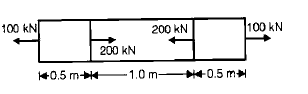Solution:
QUESTION: 5

A straight bar is fixed at edges A and B. its elastic modulus is E and cross-section is A. There is a load P = 120 N acting at C. The reactions at the ends are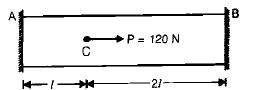Solution:
QUESTION: 6

In a tensile test, near the elastic limit zone

Solution:
QUESTION: 7

If Poisson's ratio of a material is 0.5, then the elastic modulus for the material is

Solution:
QUESTION: 8

The number of independent elastic constants required to express the stress strain relationship for a linearly elastic isotropic material is

Solution:
QUESTION: 9

The number of elastic constants for a completely anisotropic elastic material is

Solution:
QUESTION: 10

For a given material, the modulus of rigidity is 100 GPa and Poisson's ratio is 0.25. The value of modulus of elasticity in GPa is

Solution:
QUESTION: 11

The volumetric strain in case of a thin cylindrical shell of diameter 'd', thickness 't', subjected to  internal pressure 'P' is

Solution:
QUESTION: 12

The modulus of elasticity for a material is 200 GN/m2 and Poisson's ratio is 0.25. What is the modulus of rigidity ?

Solution:
QUESTION: 13

E, G, K andm represent the elastic modulus, shear modulus, Bulk modulus and poisson's ratio respectively of a linearly elastic. isotropic and homogeneous material. To express the stressstrain relations completely for this material, at  least

Solution:
QUESTION: 14

What is the relationship between the linear elastic properties; Young's modulus (E), rigidity modulus (G) and bulk modulus (K) ?

Solution:
QUESTION: 15

A copper rod 400 mm long is pulled in tension to a length of 401.2 mm by applying a tensile stress of 330 MPa. If the deformation is entirely elastic, the Young's Modulus of copper is

Solution:
QUESTION: 16

Match List – I (State of stress) with List -II (kind of loading) and select the correct answer using the codes given below the lists: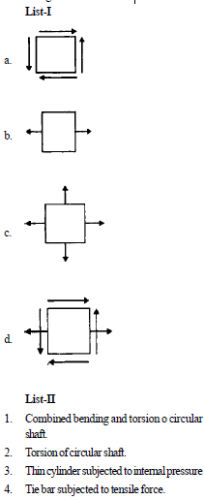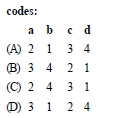Solution:
QUESTION: 17

State of stress at a point in a strained body is shown in figure A. which one of the figure given below represents correctly the mohr's circle for the state of stress?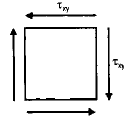Solution:
QUESTION: 18

Plane stress at a point in a body is defined by principal stresses 3s and s. The ratio of the normal stress to the maximum shear stress on the plane of maximum shear stress is

Solution:
QUESTION: 19

Which one of the following Mohr's circles represents the state of pure shear?

Solution:
QUESTION: 20

In a two dimensional problem the state of pure shear at a point is characterized by

Solution:
QUESTION: 21

A cantilever is loaded by a concentrated load P at the free and as shown. The shear stress in the element  LMNOPQRS is under consideration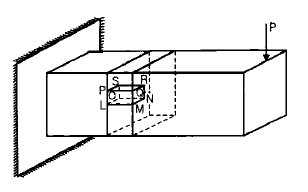Which of the following figures represents the shear stress direction in the cantilever ?

Solution:
QUESTION: 22

A circular shaft subjected to twisting moment results in maximum shear stress of 60 MPa. Then the maximum compressive stress in the material is

Solution:
QUESTION: 23

Consider a two dimensional state of stress given for an element as shown in the diagram given below: What are the coordinates of the centre of Mohr's circle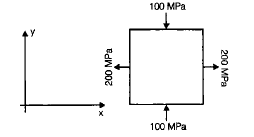Solution:
QUESTION: 24

A steel bar of 5 mm is heated from 25°C to 45°C and it is free to expand. The bar will induce

Solution:

Stress is nothing but the resisting force since due to temperature rise body will expand and if this expansion due to temperature change is restricted then only resisting stress (thermal stress) will come into play since expansion is not restricted that's why no stress is occurring.

QUESTION: 25

At a point in two-dimensional stress system sx = 100 N/mm2,sy =txy= 400 N/mm2. what drawn with a scale of 1cm = 100 N/mm2.

Solution:Use Code STAYHOME200 and get INR 200 additional OFF Use Coupon Code